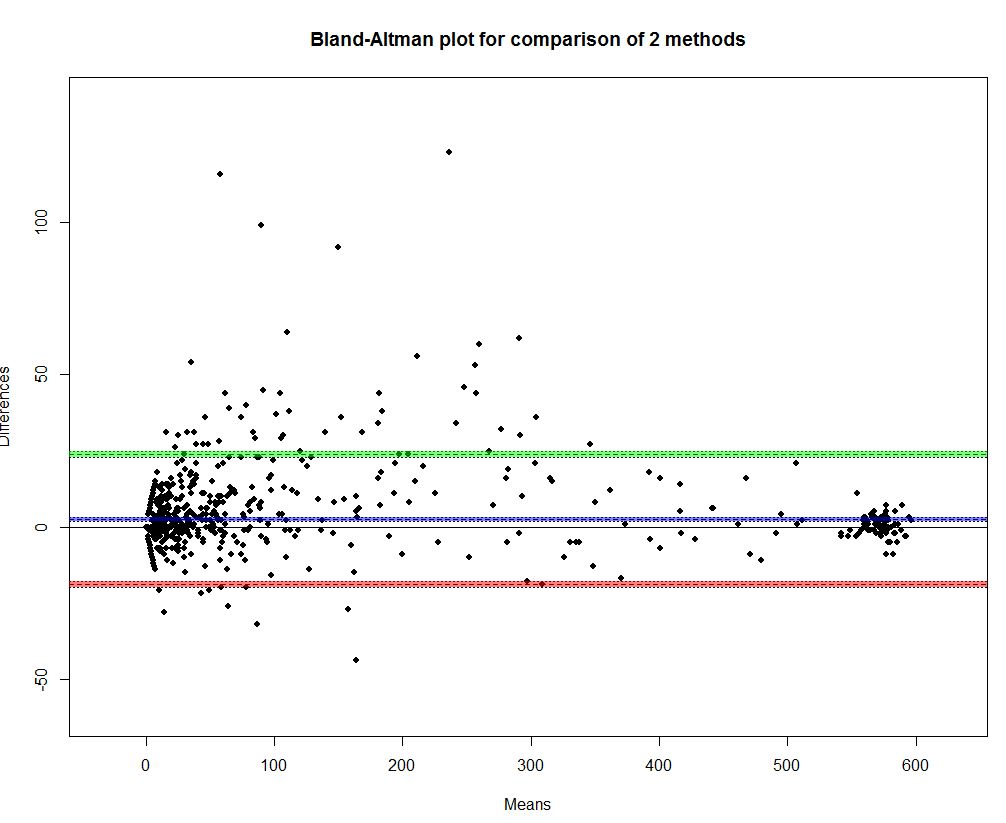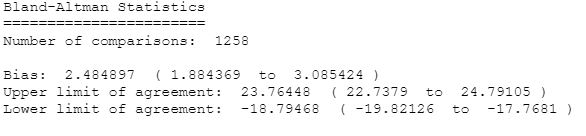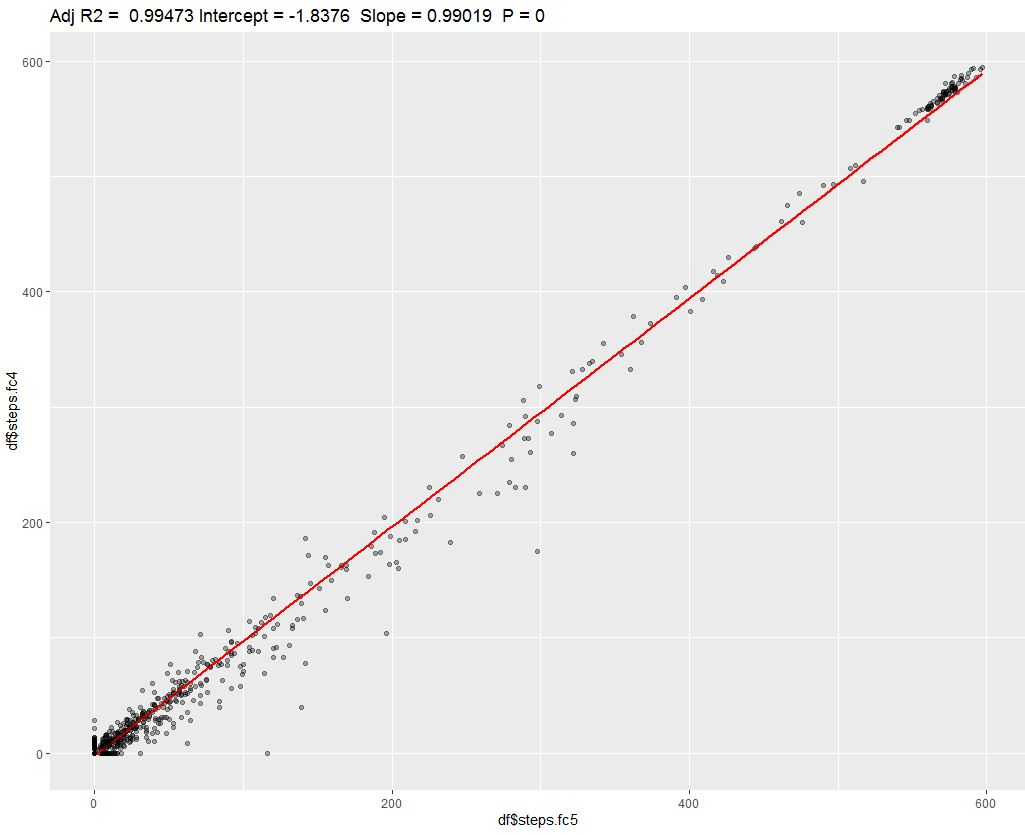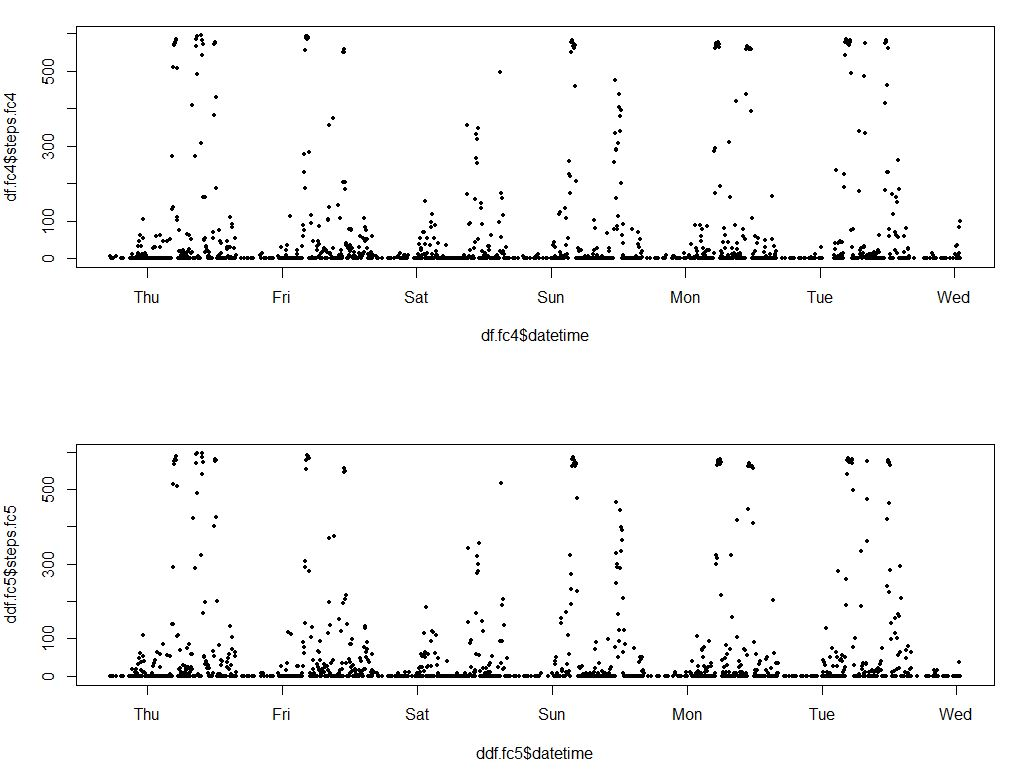Quantified Self Experiments / Accuracy - Fitbit Charge 5 vs 4 - Steps

## Abstract

#### What did i do?

I've measured my steps by using Fitbit Charge 5 and 4 simultaneously

#### How did i do it?

I've used bland altman plot approach to build agreement between 2 devices.

#### What did i learn?

Fitbit Charge 5 seems to be a biased for +2.5 steps versus Fitbit Charge 4 which is a pretty small bias. Devices agree well, with limits of agreement between -19 to +24 steps per 5 minutes. Trends are equal.

## Introduction

We can track steps to assess our physical activity which is important for general health and longevity.

The purpose of this experiment (n=1) is to compare steps data between 2 wristworn devices.

## Materials & Methods

#### Participants

Adult male anthropometrics was described in previous article.

#### Experimental design

From 2021-10-06 to 2021-10-12 Fitbit Charge 5 and Charge 4 was weared on same hand (left) and steps data were collected. There were single training session (~20 minutes of rowing) and a few ~1 hour walks.

## Results

To check steps agreement i've decided to build Bland-Altman plots. Steps were compared on same resolutions. There were total ~4700 measurements during that period.
Bland-Altman plot for 5 minute steps:X and Y axis are sum of steps for 5 minute period

How to read this plot? Imagine we have a list of 5 minute steps sums, n rows, each row contain 2 values one for FC4 and one for FC5. Then for each row we compute mean for both measures: (FC5 - FC4) / 2 and their difference (FC5 - FC4). Then we plot means on X and differences on Y.

For example, we can see that when steps per 5 minute are high (>500) devices agree well (small difference FC5 - FC4). But when there are less steps devices agree less.
Black dashed line slightly above x axis indicate bias (difference between overall means). Green and red lines represents 95% limits of agreement.Here we can see 2.5 steps bias and limits of agreement [-18.8,23.8]. Thats seems like a normal agreement

Lets build a linear regression:Adjusted R-squared is a proportion of shared variance between both devices. We can see ~99.4% value which is extrelemely good and is equal to correlation coefficient of 0.99. Devices seems to follow patterns extremely well, which is easily detected by visual inspection:## Discussion

This data analysis suggests a good agreement for absolute sum of steps for 5-min resolution window. Devices share 99.4% of variation (R-squared) and correlation coefficient is 0.99 is extrelemly large which suggests that devices follow equal trend.

## Data availability & Information

Welcome for questions, suggestions and critics in comments below.

Original unmodified (exported) raw data for Fitbit Charge 5 here and for Fitbit Charge 4 is here.

`alpha <- 0.05source("https://blog.kto.to/uploads/r/functions.R")library(jsonlite)df.fc4.raw <- na.omit(fromJSON(file.path("https://blog.kto.to/uploads/fitbit/fc4-steps-2021-10-07.json"), flatten = TRUE))df.fc5.raw <- na.omit(fromJSON(file.path("https://blog.kto.to/uploads/fitbit/fc5-steps-2021-10-05.json"), flatten = TRUE))df.fc4 <- data.frame(datetime = as.POSIXct(df.fc4.raw\$dateTime, tryFormats = c("%m/%d/%y %H:%M:%OS")), steps = as.numeric(df.fc4.raw\$value))df.fc5 <- data.frame(datetime = as.POSIXct(df.fc5.raw\$dateTime, tryFormats = c("%m/%d/%y %H:%M:%OS")), steps = as.numeric(df.fc5.raw\$value))library(lubridate)library(dplyr)df.fc4 = df.fc4 %>%  mutate(datetime = floor_date(datetime, unit = "5 minute")) %>%  group_by(datetime) %>%  summarise(steps.fc4 = sum(steps, na.rm = TRUE))df.fc5 = df.fc5 %>%  mutate(datetime = floor_date(datetime, unit = "5 minute")) %>%  group_by(datetime) %>%  summarise(steps.fc5 = sum(steps, na.rm = TRUE))par(mfrow = c(2,1))plot(df.fc4\$datetime, df.fc4\$steps.fc4, pch = 20, cex = .9)ddf.fc5 <- df.fc5[-c(1:48),]plot(ddf.fc5\$datetime, ddf.fc5\$steps.fc5, pch = 20, cex = .9)df <- left_join(df.fc4, df.fc5, by=c("datetime"))df <- na.omit(df)library(blandr)library(shiny)library(boot)blandr.statistics(df\$steps.fc5, df\$steps.fc4, sig.level = 1 - alpha, LoA.mode = 1)par(mfrow = c(1,1))blandr.draw(df\$steps.fc5, df\$steps.fc4, plotter = "rplot")#blandr.output.report(df\$steps.fc5, df\$steps.fc4)df\$diff <- df\$steps.fc5 - df\$steps.fc4summary(df\$diff); quantile(df\$diff, 0.025); quantile(df\$diff, 0.975)boot.fn <- function(data, indices) { return(mean(data[indices]))} boot_results <- boot(df\$diff, R = 1000, statistic = boot.fn); boot_resultsboot.ci(boot_results, conf=1-alpha, type="perc")lm.fit <- lm(df\$steps.fc4 ~ df\$steps.fc5)summary(lm.fit)par(mfrow = c(2,2))plot(lm.fit)library(car)par(mfrow = c(1,1))avPlots(lm.fit, ellipse = TRUE)ggplotRegression(lm.fit)`

#### Statistical analysis

RStudio version 1.3.959 and R version 4.0.2.